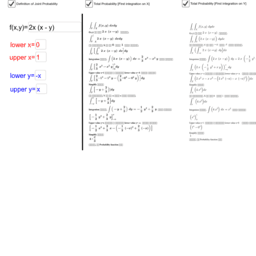# Joint Probability_Step By Step_Calculation

This GeoGebra Book helps to find double integral step by step in general, in particular, it helps to find various calculations step by step for joint probability distribution of two continuous random variable X and Y. यो GeoGebra Book ले double integral को step by step calculation हिसाब गर्न सहयोग गर्ने छ । खासगरी, यो GeoGebra Book ले दुईवटा continuous random variable X and Y को joint probability distribution मा हुने बिभिन्न calculation हरु, जस्तै Marginal probability function, Mean, Variance, Covariance आदी calculation हिसाब गर्न सहयोग गर्ने छ ।